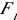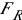## PendulumsIn the above diagram of a swinging pendulum, notice several variables that have been depicted:

a = centripetal acceleration (toward the center of the arc)

m = mass of the bob in kg

v = velocity of the bob (tangent to the circle)

W = weight of the bob in N= inertial force= component of acceleration due to gravity

The angle is with respect to the vertical.

As a pendulum swings, it undergoes an energy transformation from gravitational potential at its highest point to kinetic energy at its lowest point. Since mgh = 1/2 mv2, then v = (2gh)1/2 at the lowest point. The velocity is zero at the highest point and the height is zero at the lowest point.

The period of a pendulum is dependent on only two factors: length and acceleration due to gravity (g). The equation for the period (one complete swing back and forth) is:

T = 2 π(L/g)1/2

If you have a grandfather clock or a wall pendulum clock, you should notice that it has a movable “bob” on the end of the swinging arm. Moving this bob allows you to adjust the way time is kept on that clock…running fast or slow.

In SHM, period is sometimes used, but another term, frequency, is also used:

Whereas period is the time for one revolution; frequency is the number of cycles per second. They are reciprocals of each other. T = 1/f; f = 1/T. Period is measured in the SI unit of seconds; frequency is measured in 1/s or s-1.

(source)